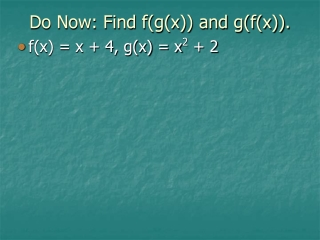DownloadDownload PresentationDo Now: Find f(g(x)) and g(f(x)).

# Do Now: Find f(g(x)) and g(f(x)).

Télécharger la présentation## Do Now: Find f(g(x)) and g(f(x)).

- - - - - - - - - - - - - - - - - - - - - - - - - - - E N D - - - - - - - - - - - - - - - - - - - - - - - - - - -
##### Presentation Transcript

1. Do Now: Find f(g(x)) and g(f(x)). • f(x) = x + 4, g(x) = x2 + 2

2. Algebra II 6.4: Use Inverse Functions HW: p.442 (4-10 even, 16, 18, 22, 26)

3. Inverse Relations • An inverse relation interchanges the input and output values (the x and y) of the original relation. • This means the domain and range also change, since the domain is your input and the range is your output.

4. Find the inverse of the relation. • y = 3x – 5 • y = 5x + ½

5. Find the inverse of the function. • f(x) = x3 – 2 • f(x)=

6. Inverse Functions • If both the relation and the inverse of the relation are functions, then they are called inverse functions.

7. Inverse functions • Functions f and g are inverses of each other provided: f (g (x ))= x and g (f (x ))= x

8. Verify that f and g are inverse functions. • f(x) = x + 4, g(x) = x – 4

9. Verify that f and g are inverse functions. • f(x) = , g(x) =

10. Find the inverse of the function. f(x) = x4 – 2 f(x)=